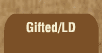Mathematics and Dyslexia

Tips for Learning the Times Tables

Tools for the Times Tables & Workbook

Home > Math > times tables practice > zeroes times tables practice

## Stage One, Level One:

No-Frills Math Times Tables Practice: Test One.

Answer these questions; when you're done, click "grade me!" and you'll find out how many you got right.

The zeroes are the easiest to remember. There are a few times tables that are infamous for being very difficult to remember. If you'd like a head start on them, try the bonus question at the end.

1. 8 x 0=
2. 1 x 0=
3. 0 x 0=
4. 9 x 0=
5. 4 x 0=
6. 24 x 0=
7. 897 x 0=
8. 5 x 0=
9. 7 x 0=
10. 2 x 0=
11. 10 x 0=
12. 3 x 0=
13. 6 x 0=
14. n x 0=
15. skunks x 0=
16. BONUS!!! 7 x 6=